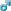Forum des amateurs de maths Vous souhaitez réagir à ce message ? Créez un compte en quelques clics ou connectez-vous pour continuer.Aide pour les futurs mathématiciensAccueilPortailRechercherS'enregistrerConnexion
Le Deal du moment :
Coffret Pokémon Ultra Premium Dracaufeu 2022 en ...

 Forum des amateurs de maths :: Mathématiques supérieurs et spéciales :: Algèbre

# joli système2 participants
AuteurMessage
belgacem
MaîtreNombre de messages : 112
Age : 59
Date d'inscription : 18/06/2012Sujet: joli systèmeMar 29 Mar 2022, 04:29naïl
MaîtreNombre de messages : 196
Age : 40
Date d'inscription : 25/04/2006Sujet: Two solutionsVen 06 Mai 2022, 15:23 (x,y,z) is a real solution to the system, then x, y and z are all positive or null because of the odd integer power 2020 in the equations. Moreovery -x = (z +x)^2020 -(y +z)^2020 = ((z +x) -(y +z))((z +x)^2019 +(z+x)^2018 (y+z) +(z+x)^2017 (y +z)² +(z +x)^2016 (y +z)^3... +(y +z)^2019) what is equivalent to (y -z)(1 +(z +x)^2019 +(z +x)^2018 (y +z)... +(z +x)^2 (y +z)^2018 +(y +z)^2019) = 0 where the second factor is positive. Then y = z. Also z = x. Finally a system equivalent is x = y = z = (2x)^2020…joli systèmePage 1 sur 1
 Sujets similaires» systeme
» Systeme
» Système.
» Un système:
» Système:

Permission de ce forum:Vous ne pouvez pas répondre aux sujets dans ce forumSauter vers: Sélectionner un forum||--Annonces - News - Les Régles à réspectées|   |--Les Régles à réspecter - Sondages|   |-- Annonces - News|   |--Partenaires|   |--Présentation des membres|   |--Préparation à l'agrégation|   |--Agrégation|   |--Concours externe|   |--Mathématiques supérieurs et spéciales|   |--Analyses|   |--Algèbre|   |--Concours|   |--Questions- Réponses|   |--Lycée|   |--Terminale|   |--Test Terminale S.M.|   |--Groupe etudiants du T S M|   |--Première|   |--Seconde - Tronc commun|   |--Préparation aux concours|   |--Collège|   |--espace aide|   |--Espace défi|   |--Olympiades|   |--Inégalités|   |--Arithmétiques|   |--Equations fonctionnelles|   |--Geométrie|   |--Combinatoire|   |--Divers|   |--théorèmes et Formules|   |--Problèmes de la semaine et du mois|   |--Problème de la semaine|   |   |--Arithmétique|   |   |--Inégalités algébriques|   |   |--Equations fonctionnelles|   |   |--Combinatoire|   |   |--Géométrie|   |   |   |--Problème du mois|   |--Histoire des Maths|   |--Histoire des Mathématiques|   |--Informatique et Physique    |--Physique    |-- logiciels de maths    |--Tutorials    |--discussion libre